# Colour Tiles

Objective: Demonstrate an understanding of addition and subtraction of decimals (tenths and hundredths), using concrete and pictorial representations.
Materials: Colour tiles, paper, pencil.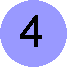Start with definitions: blue tiles represent tens, yellow tiles represent ones/units, red tiles represent tenths, green tiles represent hundredths. Try building a set of blocks which represents each of the following:     3.24  5.96  10.01  11.12  99.00  0.30 Create a page like the one shown to serve as an addition mat. To model 4.32 + 3.28, in the ones column place 4 yellow tiles, in the tenths column place 3 red tiles, and in the hundredths column place 2 green tiles. Now ... add 3 yellow tiles to the ones column, 2 red tiles to the tenths column, and 8 green tiles to the hundredths column. Regroup (remember, only a maximum of ten tiles in a given column).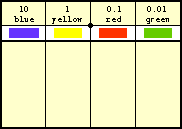Try modeling each of the following: 5.43 + 0.93   13.56 + 8.77

Objective: Recognize, model and describe multiples, factors, composites and primes.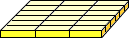Materials: Colour tiles.

 To find out if a number is prime or composite, try building arrays with colour tiles: To find out if 18 is prime or composite, take 18 colour tiles and try to arrange all 18 tiles into squares or rectangles. If the only rectangle you can make is 1 tile wide and 18 tiles long, then 18 is prime. If you can make ANY other rectangle using all the tiles, then 18 is composite. The dimensions for each rectangle built from the tiles represents the factors of the number. Likewise, the number of tiles represents a multiple of both of the dimensions of the rectangle. Determine whether each of the following numbers is prime or composite. If it is composite, make a list of all of its factors.    32   31   45   24   27    29   37   39   51   73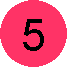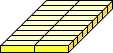Objective: Demonstrate and explain the meaning of ratio concretely, pictorially and symbolically. Demonstrate and explain the meaning of percentage concretely, pictorially and symbolically.
Materials: Colour tiles.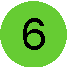To model a ratio, take the correct numbers of tiles from the box and create a set of tiles: For example ... the ratio of yellow to blue is 4 to 1 ... take out four yellow tiles and one blue tile and place them in a row. Now, add another row like the first. There is still 1 blue tile for every four yellow tiles, however we have used 10 tiles in all. Keep adding more rows and listing the equivalent ratios. To extend this to percents, simply keep adding tiles in the correct ratios until you have used 100 tiles in all (per means out of, and cent means one hundred). Now answer the questions: how many are yellow (80, thus 80%), and how many are blue (20, thus 20%). Find four equivalent ratios for each:  2 to 3   3 to 7   3 to 1 Estimate, calculate or model the percentage of each colour in the three ratios above.